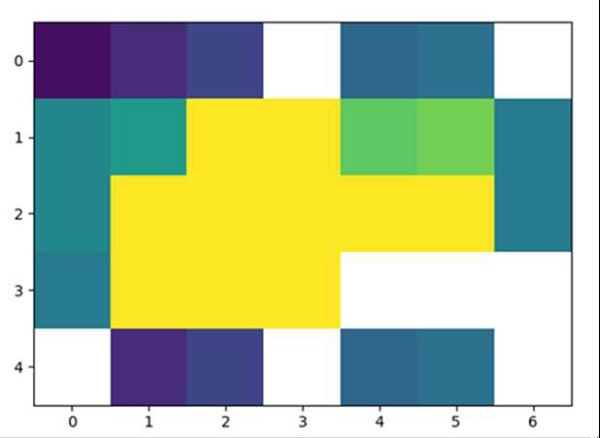# How can I plot NaN values as a special color with imshow in Matplotlib?

First, we can create an array matrix with some np.nan value, and using imshow method, we can create a diagram for that matrix.

## Steps

• Create a new figure, or activate an existing figure.

• Add an ~.axes.Axes to the figure as part of a subplot arrangement, nrows = 1, ncols = 1, index = 1.

• Create a 2D array with np.nan.

• Display data as an image, i.e., on a 2D regular raster.

• Use the draw() method which draws the drawing at the given location.

• To show the figure, use the plt.show() method.

## Example

import numpy as np
import matplotlib.pyplot as plt

f = plt.figure()
a = [
[1, 3, 5, np.nan, 8, 9, np.nan],
[11, 13, 51, 71, 18, 19, 10],
[11, 31, 51, 71, 81, 91, 10],
[10, 30, 50, 70, np.nan, np.nan, np.nan],
[np.nan, 3, 5, np.nan, 8, 9, np.nan]
]
ax.imshow(a, interpolation='nearest', vmin=0, vmax=24)
f.canvas.draw()
plt.show()

## Output